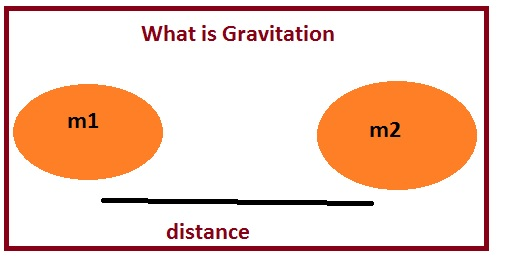Hello fellows, I hope you all are doing great. In today’s tutorial, we will have a look at Difference Between Gravitation and Gravity. Both of these terms are related to the force applying on the body existing at the surface of earth. gravitation is related with the two objects present on the earth and gravity is related with the earth and object existing on the earth.

Sometimes these terms are considered as similar but these are not alike. In today’s post, we will have a detailed look at such parameters that relate and compare gravity and gravitation. So let’s get started with Difference Between Gravitation and Gravity.

#### Difference Between Gravitation and Gravity

Gravitation

• The gravitation is the force interacting among two bodies existing in this world.
• The force between two objects can be either repulsive or attractive.
• It is a weak force of attraction and repulsion
• The gravitation force between eh gases results in the creation of a star.
• it is a universal force
• it is a week in nature
• discuss masses of objects
• To calculate this force we needed masses of two bodies that are bearing force.
• Its formula is F=Gm1m2/R2 in this equation G is gravitation constant, m1 and m2 masses of bodies and R is the distance between them.
• The value G is 6.67 x10-11 Nm2 / Kg2#### Gravity

• The force exerted by the earth on the object positioned on the surface of the earth is called gravity.
• Gravity discusses the planets stars galaxies.
• Archimedes finds the center of gravity for a triangle.
• Vitruvius tell that the gravity of the body does not rely at the weight
• Gravity is discussed through general relativity theory.
• Blackhole is an example.
• It also explained with newton law of universal gravitation
• Large size structure is based on gravity
• It has an infinite range
• Gravity tells about weight of the body and tides for moon.
• It has its own field of vector
• This force is only attractive.
• it required for explanation just mass
• This force is stronger than the gravitation force.
• It is not universal forces
• To find the value of this force we need just the mass of body placed on the surface on the earth.
• Its mathematical expression is F=mg here g is constant and m is mass of the body.
• The value of g is g = 9.8 m/s2That is detailed post about gravitation and gravity if you have any query ask in comments. Thanks for reading. Have a good day.